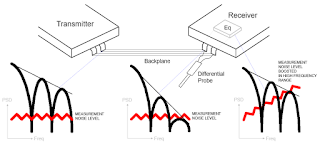## You need to test, we're here to help.

You need to test, we're here to help.

## 23 July 2018

### Feed-Forward EqualizationFigure 1: FFE creates a number of delayed versions of the input signal that are then added back to the signal with proper weights
In addition to continuous time linear equalization (CTLE), another means of improving signal quality at the receiver end of a high-speed serial data link is known as feed-forward equalization (FFE). In terms of implementation, FFE is not unlike the pre-emphasis filtering that is done on the transmitter side. An FFE implementation looks for all intents and purposes like a digital finite impulse response (FIR) filter.

FFE creates a number of delayed versions of the input signal that are added back to the signal with the proper weights (Figure 1). Typically, the delay is 1 UI in duration. FFE implementations can be done with multiple-tap filters and multiple tap coefficients, with taps being both pre-cursor and post-cursor. The weights of those taps might be positive or negative depending on whether they're pre- or post-cursor.

An important attribute of a passive equalizer such as an FFE is that the net amplitude sum of all tap values must equal 1. This is true of a pre-emphasis filter also. If the tap values do not equal 1, you'll end up with a non-linear (or non-passive) equalization.

Each stage is then added together to arrive at the output waveform. In this process, the output of each of the delay blocks is weighted and sent to a summing node. The resulting summed output is then fed to a bit slicer. This is the same process that's performed for pre-emphasis at the transmitter, but here it's being done on the received signal to compensate for pre- and post-cursor inter-symbol interference (ISI).Figure 2: These images depict serial-data signals at thetransmitter (lower left), at the receiver (lower center). andafter equalization (lower right)
Typically, FFE implementations chew up a substantial amount of power and chip real estate due to the need for multiple delay elements and tap coefficients. Thus, you'll see FFE used more often in high-cost designs where such equalization is important. Note, however, that because an FFE is performing compensation after the signal has traversed the channel, it tends to increase noise as it boosts high frequencies.

Figure 2 provides an illustration of how equalizing the measured receiver signal boosts measurement noise. At lower left is a representation of the signal's power spectral density at the transmitter that shows three lobes corresponding to the first three harmonics. At this point, the signal is easily distinguished from the oscilloscope's noise floor (in red).

That same signal, on arrival at the receiver, looks like the image at lower center, where high-frequency attenuation has taken its toll. Typically, 10-GHz frequency content can be attenuated by as much as ~60 dB, which will bury that content below the oscilloscope's measurement noise threshold.

The image at lower right of Figure 2 represents the effects of receiver equalization, which restores the high-frequency content but also boosts high-frequency noise. This is a natural consequence of the process.

Next time, we'll look at decision feedback equalization (DFE) and consider some of the limitations of the various flavors of equalization discussed previously.

Previous posts in this series:

Introduction to Debugging High-Speed Serial Links
A Look at Transmission-Line Losses
How Much Transmission-Line Loss is Too Much?
Inter-Symbol Interference (or Leaky Bits)
Rise-Time Degradation and ISI Jitter
Introduction to Channel Equalization
The Effects of De-Emphasis on Eye Diagrams
Serial-Data Channel Emulation and S Parameters
Continuous Time Linear Equalization